# Algebra II : Other Exponent Applications

## Example Questions

### Example Question #1 : Other Exponent Applications

A biologist figures that the populationof cane toads in a certain lake he is studying can be modeled by the equation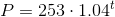,

whereis the number of days elapsed in 2015. For example,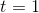represents January 1,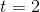represents January 2, and so forth.

If this model continues, in what month will the population of cane toads in the lagoon reach 5,000?

Possible Answers:

May

February

April

March

June

Correct answer:

March

Explanation:

Set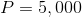and solve for: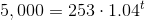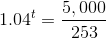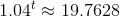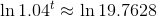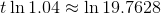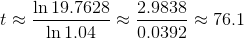January and February have 59 days total; add March, and this is 90 days. The 76th day is in March.

### Example Question #2 : Other Exponent Applications

A biologist figures that the populationof cane toads in a certain lake he is studying can be modeled by the equation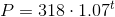,

whereis the number of days elapsed in 2015. For example,represents January 1,represents January 2, and so forth.

Assuming that this has been the model for their growth throughout the previous year as well, in what month did the population hit 100 cane toads?

Possible Answers:

August 2014

November 2014

December 2014

September 2014

October 2014

Correct answer:

December 2014

Explanation:

Set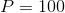and solve for: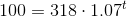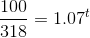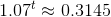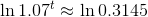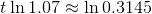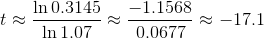17 days before January 1 was in December of 2014.

### Example Question #3 : Other Exponent Applications

Lucia deposits $40,000 into a savings account that pays 5.5% annual interest compounded continuously. Assuming she neither deposits nor withdraws money, how long will it take for her to have$60,000 in the account?

Possible Answers:

Between 9 and 10 years

Between 6 and 7 years

Between 5 and 6 years

Between 7 and 8 years

Between 8 and 9 years

Correct answer:

Between 7 and 8 years

Explanation:

We set final principal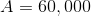original principal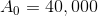, and interest rate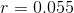. We solve forin the  continuous compound interest formula: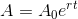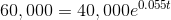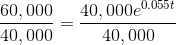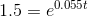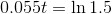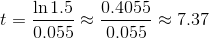The correct response is therefore between 7 and 8 years.

### Example Question #4 : Other Exponent Applications

Ann deposits $30,000 into a savings account that pays 4.5% annual interest compounded quarterly. Assuming she neither deposits nor withdraws money, what is the amount of time it will take for her to have at least$50,000 in the account?

Possible Answers: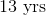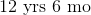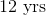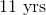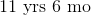Correct answer:Explanation:

Apply the compound interest formula: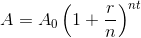.

We set final principal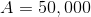original principal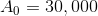, interest rate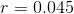, number of periods per year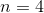(quarterly). We solve forin the equation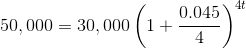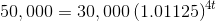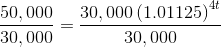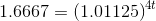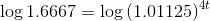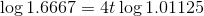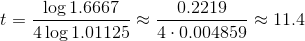This is rounded up to  the next quarter of a year, so the correct response is 11.5 years, or 11 years 6 months.

### Example Question #5 : Other Exponent Applications

A company is constructing a wall with 4-sides, all sides are of equal length.

Write an equation using exponents to calculate the area of the wall. Useas the length and height.

Possible Answers: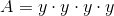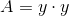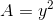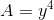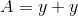Correct answer:Explanation:

The formula to find area is: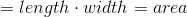is correct.

In our case our length is equal to our width which is.

Substituting our values into our equation we get: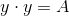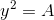### All Algebra II Resources